## Paley Construction

Hadamard Matricescan be constructed using Galois Field GF(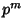) when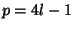andis Odd. Pick a representationRelatively Prime to. Then by coloring white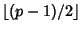(where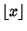is the Floor Function) distinct equally spaced Residues mod(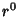,,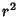, ...;,,, ...; etc.) in addition to 0, a Hadamard Matrix is obtained if the Powers of(mod) run through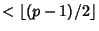. For example,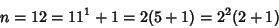is of this form withand. Since, we are dealing with GF(11), so pickand compute its Residues (mod 11), which are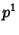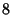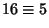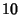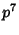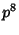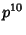Picking the firstResidues and adding 0 gives: 0, 1, 2, 4, 5, 8, which should then be colored in the Matrix obtained by writing out the Residues increasing to the left and up along the border (0 through, followed by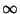), then adding horizontal and vertical coordinates to get the residue to place in each square.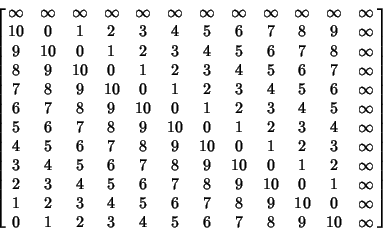can be trivially constructed from.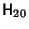cannot be built up from smaller Matrices, so use. Only the first form can be used, withand. We therefore use GF(19), and color 9 Residues plus 0 white.can be constructed from.

Now consider a more complicated case. For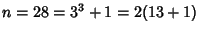, the only form havingis the first, so use the GF() field. Take as the modulus the Irreducible Polynomial, written 1021. A four-digit number can always be written using only three digits, sinceand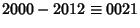. Now look at the moduli starting with 10, where each digit is considered separately. Then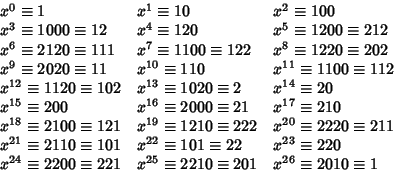Taking the alternate terms gives white squares as 000, 001, 020, 021, 022, 100, 102, 110, 111, 120, 121, 202, 211, and 221.

References

Ball, W. W. R. and Coxeter, H. S. M. Mathematical Recreations and Essays, 13th ed. New York: Dover, pp. 107-109 and 274, 1987.

Beth, T.; Jungnickel, D.; and Lenz, H. Design Theory, 2nd ed. rev. Cambridge, England: Cambridge University Press, 1998.

Geramita, A. V. Orthogonal Designs: Quadratic Forms and Hadamard Matrices. New York: Marcel Dekker, 1979.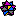Kitis, L. Paley's Construction of Hadamard Matrices.'' http://www.mathsource.com/cgi-bin/MathSource/Applications/Mathematics/0205-760.